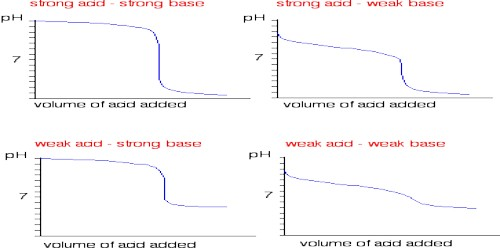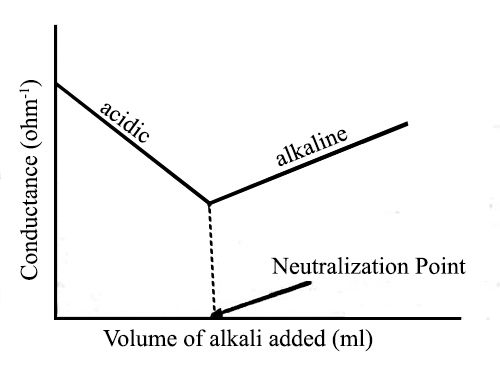Chemistry

# Conductometric Titration of Strong Acid and Strong BaseConductometric Titration of strong acid and strong base:

In a strong acid-strong base titration, the acid and base will react to form a neutral solution. The conductance of electrolytes depends on the number of ions and their speeds. For conductometric titration experiments, a known volume of the solution to be titrated is placed in a beaker and a conductivity cell dipped into it. The conductivity cell is now connected to one end of the Wheatstone’s bridge.

Strong Acid is an acid that is totally ionized in aqueous solution. This means when the strong acid is positioned in a solution such as water, all of the strong acids will detach into its ions, as contrasting to a weak acid.

Strong Base is a base that is totally ionized in aqueous solution. This means when the strong base is positioned in a solution such as water, all of the strong bases will detach into its ions.

In water, one mole of a strong acid HA dissolves yielding one mole of H+ (as hydronium ion H3O+) and one mole of the conjugate base, A.  Fundamentally, none of the non-ionized acid HA remains.

Strong acid: HA + H2O → A(aq) + H3O+(aq)

Strong base: BOH + H2O → B+(aq) + OH(aq)

An example of this would be the titration of hydrochloric acid (strong acid) and sodium hydroxide (strong base) to form sodium chloride and water.Fig: Titration of Strong acid vs Strong base

Let us consider the titration of a solution of HCl by NaOH solution. Before NaOH is added, the conductance is high due to the existence of highly mobile hydrogen ions. The net reaction in this neutralization reaction is the production of water from H+ and OH. When the base is added, the conductance falls due to the alternate of hydrogen ions by the added cation as H+ ions react with OH ions to form undissociated water. At the equivalence point, the solution contains only NaCl. The neutralization reaction can be written as-

H++ (aq) + Cl (aq) + Na+ (aq) + OH (aq) → H2O (l) + Na+ (aq) + Cl (aq)

If we cancel the common terms from both sides the net ionic reaction becomes

H+ (aq) + OH (aq) → H2O (l)

In the presence of the acid or base, all the water will remain practically undissociated. However, H+ ion of the acid is gradually replaced by Na+ as NaOH is added to the acid solution. Since the ionic conductance of H+ is much higher than that of Na+ there will be a decrease in the conductance as the addition of NaOH continues. This will go on until all the acid is neutralized. If more NaOH is added after the neutralization point, the conductance of the solution in the titration vessel will again increase as the ionic mobility of OH is very large. It the conductance values are graphically plotted against the corresponding volumes of NaOH added a conductometric titration curve is obtained [Figure]. The point of intersection of the two straight lines gives the endpoint of the titration. Thus acid-based titration can be carried out conductometrically.